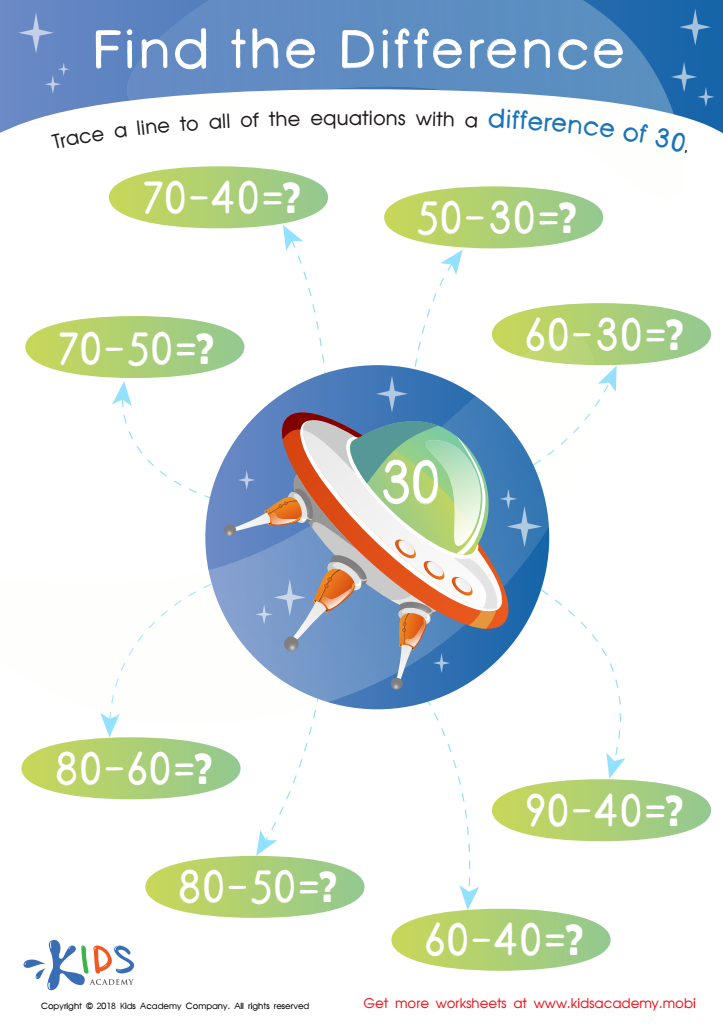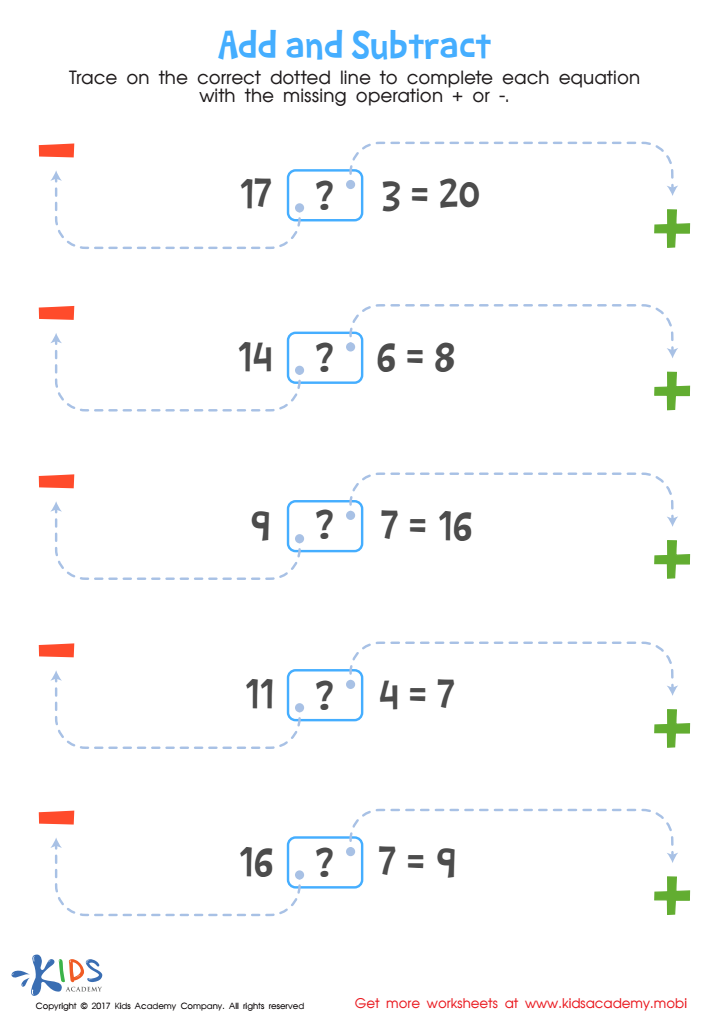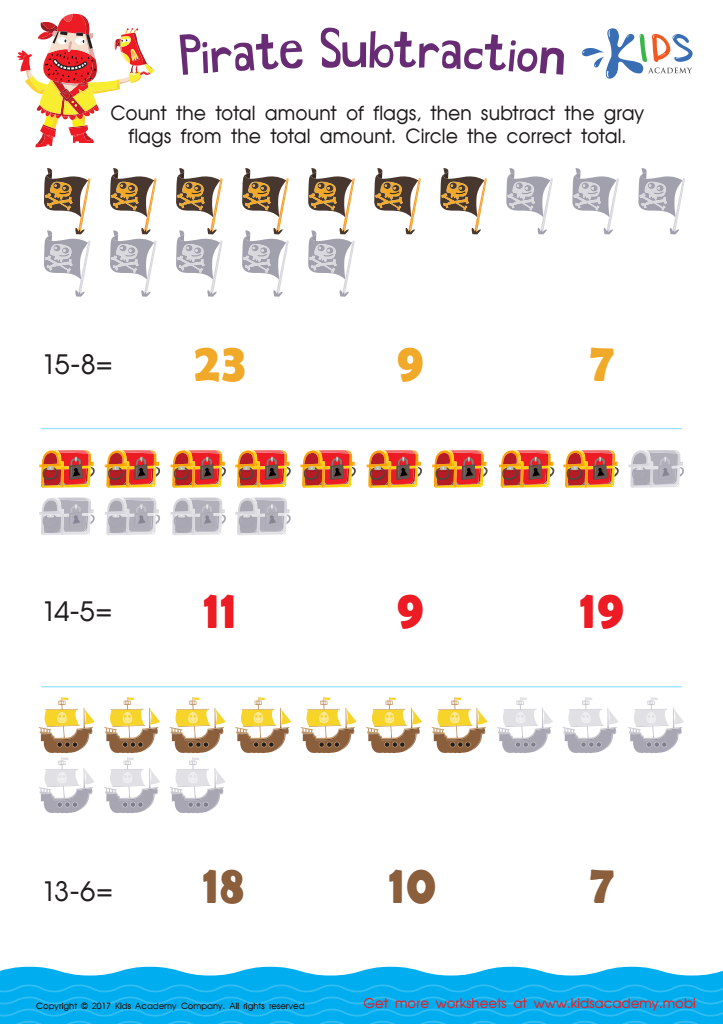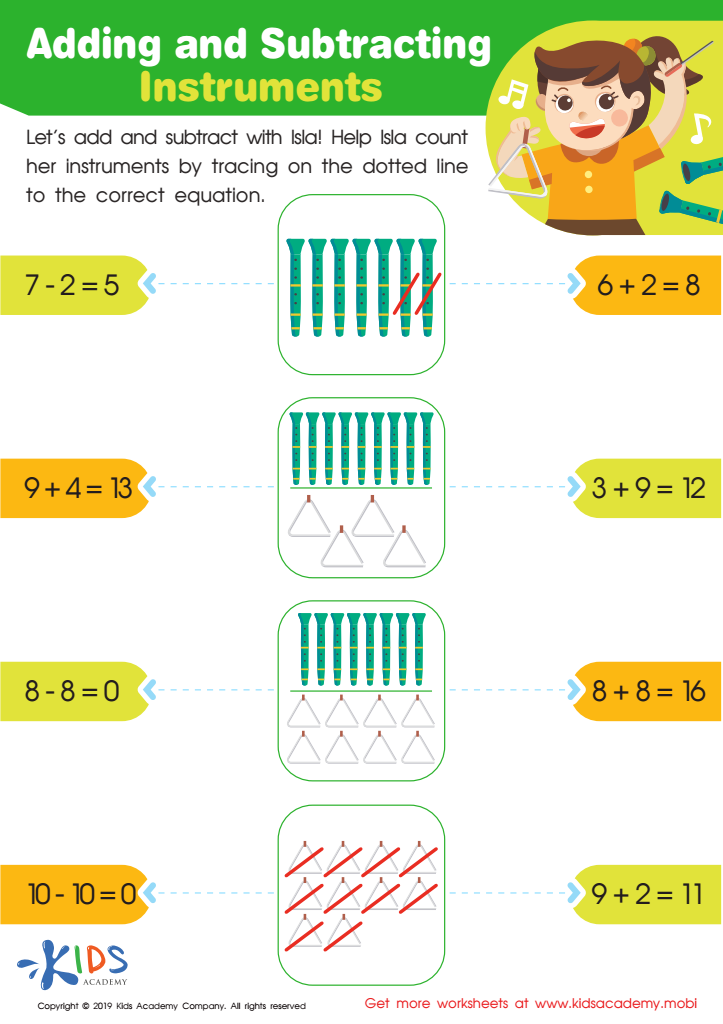# Extra Challenge Learning Subtraction Worksheets With Answer Keys for Grade 1

7 filtered results

Clear all filters
Favorites
Online only

7 filtered results

Difficulty Level

Standards

Introducing our Extra Challenge Subtraction worksheets with answers, specially designed for Grade 1 students. These engaging worksheets will provide an extra dose of excitement and learning to your classroom. Each worksheet contains an assortment of challenging subtraction problems, carefully crafted to enhance mathematical skills. With the included answer key, students can easily self-assess their progress and build confidence in their abilities. Take your students' subtraction skills to the next level with our Extra Challenge Subtraction worksheets, ensuring a solid foundation for future mathematical success.

Favorites
Online only
• Subtraction
• Extra ChallengeFind The Difference Worksheet
Kindergarteners need to work out subtraction problems and get the right answers. To help them, point to the alien ship with '30' in it on the worksheet. Get them to follow a line to the equations with a difference of 30. Solving these simple subtraction problems will give the answers.
Find The Difference Worksheet
Worksheet
Find The Difference Worksheet
Little kindergarteners must be able to correctly solve subtraction problems and arrive at the correct …
Subtraction
WorksheetIntroduce your child to equations and advanced math concepts with this worksheet - designed to help them learn add and subtract up to 20. This logical reasoning exercise will help them gain a better understanding of math, preparing them for future success. Start early and watch them excel!
Worksheet
As kids learn the basics of math, it’s important to expose them to equations early …
Subtraction
WorksheetPirate Subtraction Substraction Worksheet
Ahoy! Learn to subtract with pirate-themed math fun! This worksheet has ships and treasure chests to help your little one understand subtraction using a helpful strategy. Colorful illustrations make it a great learning experience.
Pirate Subtraction Substraction Worksheet
Worksheet
Pirate Subtraction Substraction Worksheet
Ahoy, matey! Let’s learn to subtract the pirate way, with this fun-filled math worksheet: pirate …